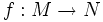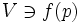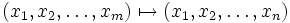# Local submersion theorem

Let$M$ and$N$ be differential manifolds of dimensions$m > n$. Let$f: M \to N$ be a differentiable map from$M$ to$N$. Let$p$ be a point such that$f$ is a submersion at$p$, i.e. the induced map on tangent spaces is surjective at$p$. Then, there exists a neighbourhood$U \ni p$ in$M$ and$V \ni f(p)$ in$N$ and coordinate charts for$U$ and$V$ such that in those coordinate charts, the map is a projection map:$(x_1, x_2, \ldots, x_m) \mapsto (x_1, x_2, \ldots, x_n)$